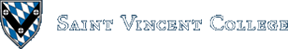Computing & Information Systems    DepartmentSearch CIS Site      Tutorials

## Software Design Using C++

### Loop Properties

The following summarizes some of the basic properties of loops in C++. This can be useful in deciding which loop to use and in avoiding problems in coding. See also the Programming Patterns section for some commonly-used patterns involving loops.
 Number of times to do the loop body must be known in advance? WHILE loop: no DO..WHILE loop: no FOR loop: no A FOR loop in C++ can do anything the WHILE loop can; it is very general. However, the answer is yes for a straightforward counting loop. Here is an example of how such a counting loop might be set up. Note that the `Count` must be known ahead of time: ```for (k = 0; k < Count; k++)    cout << k << endl;``` Minimum number of times that the loop body is executed: WHILE loop: 0 DO..WHILE loop: 1 This is because the condition is tested at the bottom of the loop. FOR loop: 0 Can give an infinite loop? WHILE loop: yes DO..WHILE loop: yes FOR loop: yes However, if set up correctly as a straightforward counting loop the answer is no. See above for an example of such a counting loop. (Note that it is important that the loop body does not modify `k`, the loop control variable. For example, if we changed this example so that the loop body decremented `k` by 1, using a statement such as `k--`, then overall the value of k would not change and we would have an infinite loop. In effect, the `k--` and the `k++` would cancel each other out.

### Related Items

Back to the main page for Software Design Using C++

Author: Br. David Carlson with contributions by Br. Isidore Minerd
Last updated: August 27, 2009
Disclaimer